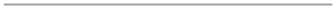Robotics 2
Problem Solving and Searching
QuizQuestion 3:  In general, which kind of algorithm will work better, informed or uninformed?
Question 9: What is one major drawback of the breadth-first algorithm?
Question 10: Which of these equations is used in the A* algorithm?
Question 2: Which of these describes the meaning of 'problem space'?
Question 1:  Which of the following describes the difference between an informed algorithm and an uninformed algorithm?
An uninformed algorithm requires that the robot must make all of its decisions first, then make all of its actions, while an informed algorithm makes decisions and takes actions at the same time.
An informed algorithm requires that the robot must make all of its decisions first, then make all of its actions, while an uninformed algorithm makes decisions and takes actions at the same time.
If we use an informed algorithm, then the robot will always know or be able to estimate the distance between its current state and the goal state.  If we use an uninformed algorithm, then the robot won't be able to know or estimate the distance.
An informed algorithm will only work if we can know or estimate the distance between the robot's current state and the goal state, while an uninformed algorithm doesn't require this information
Problem space is a representation of the problem that includes all of the actions the robot can take, and all of the states that will result from the actions.
Problem space is a grid, like a room, that the robot can move through from point A to point B.
Problem space is a tree representation of all of the barriers in the room.
Problem space is a grid that illustrates all of the actions that the robot can take.
Informed
UninformedQuestion 4:  Which of the following is true of informed search algorithms
An informed search algorithm won't work unless the robot knows the distance from its current state to the goal stateQuestion 5:  Which of these describes the difference between an online search method and an offline search method?Questions 6-8:  Characterize each of the search methods we learned about today in terms of whether they are online or offline, and informed or uninformed
An informed search algorithm will work as long as it is possible to estimate the distance from the robot's current state to the goal state
An online algorithm requires that the robot must make all of its decisions first, then make all of its actions, while an offline algorithm makes decisions and takes actions at the same time.
An offline algorithm requires that the robot must make all of its decisions first, then make all of its actions, while an online algorithm makes decisions and takes actions at the same time.
If we use an online algorithm, then the robot will always know or be able to estimate the distance between its current state and the goal state.  If we use an offline algorithm, then the robot won't be able to know or estimate the distance.
An online algorithm will only work if we can know or estimate the distance between the robot's current state and the goal state, while an offline algorithm doesn't require this informationOnline
Offline
Informed
UninformedThe breadth-first algorithm is very computationally-intensive
The breadth-first algorithm requires the robot to know (or at least estimate) the distance between its current state and the goal state.
The breadth-first algorithm is not complete
The breadth-first algorithm does not find the shortest path to the goal state
Score(Action) = Cost(Action) + Distance from State to Goal State
Score(Action) = Cost(Action) - Distance from State to Goal State
Score(Action) = Cost(Action) + Distance from State to Barrier State
Score(Action) = Cost(Action) - Distance from State to Barrier State